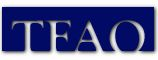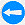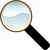The TFAO Digital Library

On 10/10/21 the online TFAO Digital Library had these file counts:

Text:

1aa = 701
2aa = 687
3aa = 705
4aa = 706
5aa = 683
6aa = 693
7aa = 562
8aa = 688
9aa = 671
10aa = 689
11aa = 435

apprais = 160
andres = 8
anne = 12
states = 7
beserra = 32
subjects = 57
book = 3
bookpage = 172
calendar = 50
chronology = 8
distingu = 55
msjc = 8
newsassn = 76
newsm1 = 703
newsmu = 669
permc = 58
srcbook = 18
utah = 10
site = 44

text total = 9,400

Image:

1am = 641
2am = 629
3am = 699
4am = 698
5am = 698
6am = 698
7am = 695
8am = 687
9am = 631
10am = 683
11am = 684
12am = 685
13am = 677
14am = 686
15am = 244
16am = 678
17am = 685
18am = 368
19am = 117

am series subtotal = 11,583

1cm = 799
2cm = 727
3cm = 698
4cm = 895
5cm = 667
6cm = 638
7cm = 695
8cm = 528
9cm = 697
10cm = 349

cm series subtotal = 6,731

okeeffe = 14
permc = 67
srcbook = 30
utah = 11
canyon = 36
distingu = 453
enman = 15
logo = 118
lrsmith = 17
mdixon = 24
mi = 719
mi1 = 36
mn = 697
mia = 241
mib = 450
mic = 499
mid = 132
msjc = 18
musimage = 698
newsassn = 110
nmtrip = 12
apprais = 144
book = 56

miscellaneous subtotal = 4,593

image total = 22,907

Grand total of text and image files = 32,307Return to Content and paper-printed book equivalence.

Go toSearch Resource Library